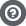Hello Guest

# 2D Rotation Problem

• 4 Replies
• 2752 Views####Yannick

•• 3##### 2D Rotation Problem
« on: March 29, 2016, 12:00:00 »
Hi guys,

I got a problem with i guess it's my transformation matrix. Every time i'm trying to totate a simple rectangle it is being bent. I am new to this lwjgl and opengl, but i think the problem lies in the tansformation matrix.
Code: [Select]
`public static Matrix4f createTransformationMatrix(Vector2f translation, Vector2f scale, float rotation) { Matrix4f matrix = new Matrix4f(); matrix.setIdentity(); Matrix4f.translate(translation, matrix, matrix); Matrix4f.rotate((float) Math.toRadians(rotation), new Vector3f(0,0,1), matrix, matrix); Matrix4f.scale(new Vector3f(scale.x, scale.y, 0 ), matrix, matrix); return matrix; }`I apologize in advance if it's a really stupid misstake.##### Re: 2D Rotation Problem
« Reply #1 on: March 29, 2016, 18:40:57 »
There is nothing wrong with that code. It produces a correct matrix given that your 'scale' parameter uses the same value for both its x and y components. You seem to not account for your window's aspect ratio in your projection matrix, so that it *seems* that this rectangle (or rather square) gets bent along the longer of the two window axes when it rotates.

If you are using the fixed-function pipeline in OpenGL, then this will help:
Code: [Select]
`static void setupProjectionMatrix() { int width = Display.getWidth(); int height = Display.getHeight(); float aspect = (float)width/height; int mode = GL11.glGetInteger(GL11.GL_MATRIX_MODE); GL11.glMatrixMode(GL11.GL_PROJECTION); GL11.glLoadIdentity(); GL11.glOrtho(-aspect, aspect, -1, 1, -1, 1); GL11.glMatrixMode(mode);}`
« Last Edit: March 29, 2016, 18:51:13 by Kai »####Yannick

•• 3##### Re: 2D Rotation Problem
« Reply #2 on: March 29, 2016, 21:55:58 »
Thanks, that indeed was the problem. I fixed it through simply scaling against the ratio problem before rotation:
Code: [Select]
`public static Matrix4f createTransformationMatrix(Vector2f translation, Vector2f scale, float rotation) { Matrix4f matrix = new Matrix4f(); matrix.setIdentity(); Matrix4f.translate(translation, matrix, matrix); Matrix4f.scale(new Vector3f(1, 1f* Display.getWidth()/Display.getHeight(), 0), matrix, matrix); Matrix4f.rotate((float) Math.toRadians(rotation), new Vector3f(0, 0, 1), matrix, matrix); Matrix4f.scale(new Vector3f(scale.x, scale.y, 0), matrix, matrix); return matrix; }`##### Re: 2D Rotation Problem
« Reply #3 on: March 30, 2016, 08:06:52 »
Just note that with a correct projection matrix the aspect ratio scaling is actually multiplied _before_ your own matrix transformations.
If you multiply the scaling after the translation, like you did, you'll notice that no matter how wide or tall your window gets resized, its corners will always be at -1 and +1 in X and Y. So you are basically stretching the coordinate system, and to counter that you squeeze your quad. So the following will give consistent behaviour which will make the window/viewport be a "window into a 2D coordinate system", with uniform scaling:
Code: [Select]
`static Matrix4f createTransformationMatrix(Vector2f translation, Vector2f scale, float rotation) {    Matrix4f matrix = new Matrix4f();    matrix.setIdentity();    Matrix4f.scale(new Vector3f((float)Display.getHeight()/Display.getWidth(), 1, 0), matrix, matrix);    Matrix4f.translate(translation, matrix, matrix);    Matrix4f.rotate((float) Math.toRadians(rotation), new Vector3f(0, 0, 1), matrix, matrix);    Matrix4f.scale(new Vector3f(scale.x, scale.y, 0), matrix, matrix);    return matrix;}`####Yannick

•• 3##### Re: 2D Rotation Problem
« Reply #4 on: March 30, 2016, 12:51:58 »
Now it's working perfect, thanks